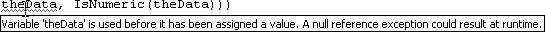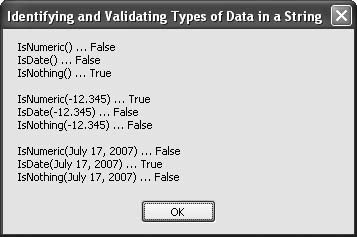# Recipe 5.22. Identifying and Validating Types of Data in a String

#### Problem

You want to check a string variable to see whether it has been assigned a value, or if it can be converted to a number, date, or time. This check can prevent an exception, and it can free your code from having to use an exception as part of its testing logic.

#### Solution

Sample code folder: Chapter 05\StringTypes

Visual Basic 2005 has three string functions that help solve this problem: IsNothing(), IsNumeric(), and IsDate(). Use these to test a string's contents before attempting conversions.

#### Discussion

The following code demonstrates the use of these three functions with data set to Nothing:

` Dim theData As String = Nothing Dim result As New System.Text.StringBuilder ' ----- Format nothing. result.AppendLine(String.Format( _    "IsNumeric({0}) … {1}", theData, IsNumeric(theData))) result.AppendLine(String.Format( _    "IsDate({0}) … {1}", theData, IsDate(theData))) result.AppendLine(String.Format( _    "IsNothing({0}) … {1}", theData, IsNothing(theData))) result.AppendLine() `

String variables are normally undefined, assigned the value of Nothing. We specifically assigned theData the value Nothing in the above code, but if we had left it blank Visual Studio would have questioned our motives and marked the first use of theData with a warning, as shown in Figure 5-24. As you can see, the unassigned string variable has squiggly lines under it, indicating a problem; hovering the mouse pointer over it causes the displayed explanation to pop up. This is a nonfatal warning, and the program will still run.

##### Figure 5-24. Visual Studio warns you if you attempt to use a string that has no data assigned to itAs shown in the first three lines of output displayed in Figure 5-25 (below), in this case the IsNumeric() and IsDate() functions verify that the string does not represent a valid number or date, but it does pass the IsNothing() test, as expected.

Next, the string is assigned a value that represents a valid number:

` ' ----- Format a number in a string. theData = "-12.345" result.AppendLine(String.Format( _    "IsNumeric({0}) … {1}", theData, IsNumeric(theData))) result.AppendLine(String.Format( _    "IsDate({0}) … {1}", theData, IsDate(theData))) result.AppendLine(String.Format( _    "IsNothing({0}) … {1}", theData, IsNothing(theData))) result.AppendLine() `

When the three tests are repeated, they match expectations. As shown in the middle three lines of output in Figure 5-25, the IsNumeric() test now returns TRue, and the IsDate() and IsNothing() tests return False.

Finally, the string is assigned a valid date, and the three tests are repeated for the last time:

` ' ----- Format a date in a string. theData = "July 17, 2007" result.AppendLine(String.Format( _    "IsNumeric({0}) … {1}", theData, IsNumeric(theData))) result.AppendLine(String.Format( _    "IsDate({0}) … {1}", theData, IsDate(theData))) result.Append(String.Format( _    "IsNothing({0}) … {1}", theData, IsNothing(theData))) MsgBox(result.ToString()) `

In this last case the IsDate() function returns true, and the other two tests return False, as shown in the last three lines of output in Figure 5-25.

Recipes 5.24 and 5.25 show how to examine content for correct processing.

##### Figure 5-25. Results of testing a string's contentsVisual Basic 2005 Cookbook: Solutions for VB 2005 Programmers (Cookbooks (OReilly))
ISBN: 0596101775
EAN: 2147483647
Year: 2006
Pages: 400

Similar book on Amazon